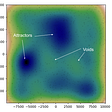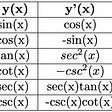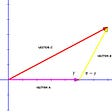# The Importance of Different Coordinate Systems

Though most of us are familar with Cartesian coordinates, some quantum mechanical problems simplify greatly if calculated in a different coordinate system. The three main coordinate systems for modeling objects in three dimensions are Cartesian, spherical, and cyclindrical. This article will give an overview of these three systems and show the equations that are needed to convert between the systems.

# Cartesian Coordiantes

Cartesian coordinates are the coordinate system you are likely most familar with. The cartesian coordinate system is based in three straight axes: x, y, and z. These three axes form three planes: the xy plane, the xz plane, and the yx plane. A point in cartesian space is simple represented as a string of three numbers, (x, y, z), where each number is the distance the point is from the origin, in the direction of the respective axis. The variables x, y, and z can be positive or negative depending on which side of each axis the point is on. In two dimensions, these coordinates are still referred to as Cartesian coordinates and typically the problem is limited to the xy plane. Cartesian coordinates work well for problems that have plane symmetry and are used often due to familarity.

# Spherical Coordinates

Spherical coordiantes describe points as if they are located on the surface of a sphere. Spherical coordinates consist of three variables: r, φ, and θ. The variable r, or sometimes ρ, is called the radial distance and is a measure of how far a point is from the origin. The variable φ is called the polar angle and is a a measure of the angle between the point and the positive x axis. Finally θ is known as the azimuthal angle and is a measure of the angle between the point and the positive z axis. Spherical coordinates work well for describing systems with spherical symmetry or when describing spherical or circular motion. They are frequently used in astronomy among other fields. In quantum mechanics these are seen, among other places, in spherical harmonics and angular momentum.

In two dimensions, limited to just the xy plane, spherical coordinates are typically referred to as polar coordinates. In polar coordintes r refers to the distance from the origin and θ to the angle from the positive x axis. Polar coordinates work well with a two dimensional system that has angualr or radial symmetry.

Note that some texts, particularly from mathemtics, may switch the definitions of θ and φ, so be careful when using a new resource.

# Cylindrical Coordinates

Finally, we have cylindrical coordinates, which combines aspects of both Cartesian and spherical coordinates. The cyclindrical coordianate system describes points as if they were on the top edge of a cylinder. The radial distance ρ, or sometimes r, and the polar angle φ have the same definitions as they do in spherical coordinates, while the third coordinate in cylindrical coordinates is z, which has the same definition as it does in Cartesian coordinates. Cyclinderical coordinates in two-dimensions would also become polar cooordinates. These coordinates are useful when a problem has cyclindrical symmetry. For example, they are often seen in electromagnetism when modeling electric and magnetic fields around wires.

# Switching Between Coordinate Systems

## Cartesian to Spherical

For φ, be careful that the formula is putting φ in the correct quadrant and be careful when the inverse tangent function is undefined.

## Cartesian to Cylindrical

For φ, be careful that the formula is putting φ in the correct quadrant and be careful when the inverse tangent function is undefined.

## Cylindrical to Cartesian

For converting from spherical to cyclinderical or vice versa, I would recommend going through Cartesian coordinates, just to minimize the number of equations that you will need to memorize or keep on hand.

Follow for more articles on physics, mathematics, and programming!

--

--

--

## More from Julie Butler Hartley

Graduate student studying theoretical nuclear physics and machine learning

Love podcasts or audiobooks? Learn on the go with our new app.

## The t-distribution: a key statistical concept discovered by a beer brewery## Known since Antiquity: The Platonic Solids## Abstract Algebra: Seeing the Same Thing in Different Places## Boating While InfraRed Imaging? BWI…RI?## The Monty Hall Problem for Dummies## Randonauting for Dummies: How to hack reality with your phone using Quantum Randomness## Julie Butler Hartley

Graduate student studying theoretical nuclear physics and machine learning

## The Opticks of Super Vision — Explaining the Double-Slit Experiment## Mathematics for Quantum Mechanics: Derivatives of a Single Variable## A Geometric Approach to the Quantum Interference Term## Part 4: Determinants